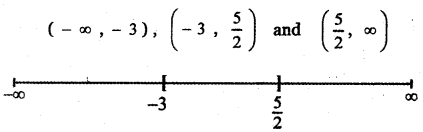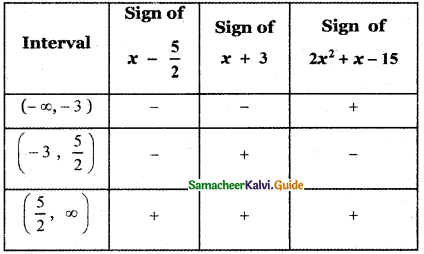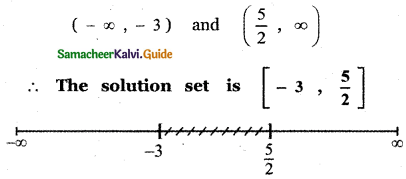Tamilnadu State Board New Syllabus Samacheer Kalvi 11th Maths Guide Pdf Chapter 2 Basic Algebra Ex 2.5 Text Book Back Questions and Answers, Notes.

## Tamilnadu Samacheer Kalvi 11th Maths Solutions Chapter 2 Basic Algebra Ex 2.5

Question 1.
Solve 2x2 + x – 15 ≤ 0
The given inequality is
2x2 + x – 15 ≤ 0 ——— (1)
2x2 + x – 15 = 2x2 + 6x – 5x – 15
= 2x (x + 3) – 5 (x + 3)
= (2x – 5)(x + 3)
2x2 + x – 15 = 2$$\left(x-\frac{5}{2}\right)$$)(x + 3) ——— (2)
The critical numbers are x – $$\frac{5}{2}$$ = 0 or x + 3 = 0
The critical numbers are x = $$\frac{5}{2}$$ or x = – 3
Divide the number line into three intervals(i) (- ∞, – 3)
When x < – 3 say x = – 4
The factor x – $$\frac{5}{2}$$ = – 4 – $$\frac{5}{2}$$ < 0 and
x + 3 = – 4 + 3 = – 1 < 0
x – $$\frac{5}{2}$$ < 0 and x + 3 < 0
⇒ $$\left(x-\frac{5}{2}\right)$$ (x + 3) > 0
Using equation (2) 2x2 + x – 15 > 0
∴ 2x2 + x – 15 ≤ 0 is not true in (- ∞, – 3)

(ii) $$\left(-3, \frac{5}{2}\right)$$
When – 3 < x < $$\frac{5}{2}$$ say x = 0
The factor x – $$\frac{5}{2}$$ = 0 – $$\frac{5}{2}$$ = – $$\frac{5}{2}$$ < 0 and
x + 3 = 0 + 3 = 3 > 0
x – $$\frac{5}{2}$$ < 0 and x + 3 > 0
⇒ $$\left(x-\frac{5}{2}\right)$$ (x + 3) < 0
using equation (2) 2x2 + x – 15 < 0
∴ 2x2 + x – 15 ≤ 0 is true in $$\left(-3, \frac{5}{2}\right)$$(iii) $$\left(\frac{5}{2}, \infty\right)$$
When x > $$\frac{5}{2}$$ say x = 3
The factor x – $$\frac{5}{2}$$ = 3 – $$\frac{5}{2}$$ > 0 and
x + 3 = 3 + 3 > 0
x – $$\frac{5}{2}$$ > 0 and x + 3 > 0
= $$\left(x-\frac{5}{2}\right)$$ (x + 3) > 0
Using equation (2) 2x2 + x – 15 > 0
∴ 2x2 + x – 15 ≤ 0 is not true in $$\left(\frac{5}{2}, \infty\right)$$We have proved the inequality 2x2 + x – 15 ≤ 0 is true in the interval $$\left(-3, \frac{5}{2}\right)$$
But it is not true in the intervalInequality solver that solves an inequality with the details of the calculation: linear inequality, quadratic inequality.

Question 2.
Solve x2 + 3x – 2 ≥ 0
The given inequality is
– x2 + 3x – 2 ≥ 0
x2 – 3x + 2 < 0 ——– (1)
x2 – 3x + 2 = x2 – 2x – x + 2
= x(x – 2) – 1(x – 2)
x2 – 3x + 2 = (x – 1) (x – 2) ——— (2)
The critical numbers are
x – 1 = 0 or x – 2 = 0
The critical numbers are
x = 1 or x = 2
Divide the number line into three intervals
(- ∞, 1), (1, 2) and (2, ∞).(i) (- ∞, 1)
When x < 1 say x = 0
The factor x – 1 = 0 – 1 = – 1 < 0 and
x – 2 = 0 – 2 = – 2 < 0
x – 1 < 0 and x – 2 < 0
⇒ (x – 1)(x – 2) > 0
Using equation (2) x2 – 3x + 2 > 0
∴ The inequality x2 – 3x + 2 ≤ 0 is not true in the interval (- ∞, 1 )(ii) (1, 2)
When x lies between 1 and 2 say x = $$\frac{3}{2}$$
The factor x – 1 = $$\frac{3}{2}$$ – 1 = $$\frac{1}{2}$$ > 0 and
x – 2 = $$\frac{3}{2}$$ – 2 = – $$\frac{1}{2}$$ – < 0
x – 1 > 0 and x – 2 < 0
⇒ (x – 1)(x – 2) < 0
Using equation (2) x2 – 3x + 2 < 0
∴ The inequality x2 – 3x + 2 ≤ 0 is true in the interval (1, 2 )(iii) (2, ∞)
When x > 2 say x = 3
The factor x – 1 = 3 – 1 = 2 > 0 and
x – 2 = 3 – 2 = 1 > 0
x – 1 > 0 and x – 2 > 0
= (x – 1)(x – 2) > 0
Using equation (2) x2 – 3x + 2 > 0
∴ The inequality x2 – 3x + 2 ≤ 0 is not true in the interval (2, ∞)
We have proved the inequality x2 – 3x + 2 ≤ 0 is true in the interval [ 1, 2 ].
But it is not true in the interval
(- ∞, 1) and (2, ∞)
∴ The solution set is [1, 2]